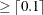### Example 5.8 A Simple Integer Program

Recall the linear programming problem presented in Chapter 3: Introduction to Optimization in SAS/OR 12.3 User's Guide: Mathematical Programming. In that problem, a firm produces two products, chocolates and gumdrops, that are processed by four processes: cooking, color/flavor, condiments, and packaging. The objective is to determine the product mix that maximizes the profit to the firm while not exceeding manufacturing capacities. The problem is extended to demonstrate a use of integer-constrained variables.

Suppose that you must manufacture only one of the two products. In addition, there is a setup cost of 100 if you make the chocolates and 75 if you make the gumdrops. To identify which product will maximize profit, you define two zero-one integer variables, `ICHOCO` and `IGUMDR`, and you also define two new constraints, `CHOCOLATE` and `GUM`. The constraint labeled `CHOCOLATE` forces `ICHOCO` to equal one when chocolates are manufactured. Similarly, the constraint labeled `GUM` forces `IGUMDR` to equal 1 when gumdrops are manufactured. Also, you should include a constraint labeled `ONLY_ONE` that requires the sum of `ICHOCO` and `IGUMDR` to equal 1. (Note that this could be accomplished more simply by including `ICHOCO` and `IGUMDR` in a SOSEQ set.) Since `ICHOCO` and `IGUMDR` are integer variables, this constraint eliminates the possibility of both products being manufactured. Notice the coefficients -10000, which are used to force `ICHOCO` or `IGUMDR` to 1 whenever `CHOCO` and `GUMDR` are nonzero. This technique, which is often used in integer programming, can cause severe numerical problems. If this driving coefficient is too large, then arithmetic overflows and underflow may result. If the driving coefficient is too small, then the integer variable may not be driven to 1 as desired by the modeler.

The objective coefficients of the integer variables `ICHOCO` and `IGUMDR` are the negatives of the setup costs for the two products. The following is the data set that describes this problem and the call to PROC LP to solve it:

```data;
format _row_ \$10. ;
input _row_ \$ choco gumdr ichoco igumdr _type_ \$ _rhs_;
datalines;
object        .25    .75   -100    -75 max        .
cooking        15     40      0      0 le     27000
color           0  56.25      0      0 le     27000
package     18.75      0      0      0 le     27000
condiments     12     50      0      0 le     27000
chocolate       1      0 -10000      0 le         0
gum             0      1      0 -10000 le         0
only_one        0      0      1      1 eq         1
binary          .      .      1      2 binary     .
;
```
```proc lp;
run;
```

The solution shows that gumdrops are produced. See Output 5.8.1.

Output 5.8.1: Summaries and an Integer Programming Iteration Log

The LP Procedure

Problem Summary
Objective Function Max object
Rhs Variable _rhs_
Type Variable _type_
Problem Density (%) 25.71

Variables Number

Non-negative 2
Binary 2
Slack 6

Total 10

Constraints Number

LE 6
EQ 1
Objective 1

Total 8

Integer Iteration Log
Iter Problem Condition Objective Branched Value Sinfeas Active Proximity
1 0 ACTIVE 397.5 igumdr 0.9 0.2 2 .
2 1 SUBOPTIMAL 260 . . . 1 70
3 -1 SUBOPTIMAL 285 . . . 0 .

Solution Summary

Integer Optimal Solution
Objective Value 285

Phase 1 Iterations 0
Phase 2 Iterations 5
Phase 3 Iterations 5
Integer Iterations 3
Integer Solutions 2
Initial Basic Feasible Variables 9
Time Used (seconds) 0
Number of Inversions 5

Epsilon 1E-8
Infinity 1.797693E308
Maximum Phase 1 Iterations 100
Maximum Phase 2 Iterations 100
Maximum Phase 3 Iterations 99999999
Maximum Integer Iterations 100
Time Limit (seconds) 120

Variable Summary
Col Variable Name Status Type Price Activity Reduced Cost
1 choco DEGEN NON-NEG 0.25 0 0
2 gumdr BASIC NON-NEG 0.75 480 0
3 ichoco DEGEN BINARY -100 0 0
4 igumdr   BINARY -75 1 -2475
5 cooking BASIC SLACK 0 7800 0
6 color   SLACK 0 0 -0.013333
7 package BASIC SLACK 0 27000 0
8 condiments BASIC SLACK 0 3000 0
9 chocolate   SLACK 0 0 -0.25
10 gum BASIC SLACK 0 9520 0

Constraint Summary
Row Constraint Name Type S/S Col Rhs Activity Dual Activity
1 object OBJECTVE . 0 285 .
2 cooking LE 5 27000 19200 0
3 color LE 6 27000 27000 0.0133333
4 package LE 7 27000 0 0
5 condiments LE 8 27000 24000 0
6 chocolate LE 9 0 0 0.25
7 gum LE 10 0 -9520 0
8 only_one EQ . 1 1 2400

The branch-and-bound tree can be reconstructed from the information contained in the integer iteration log. The column labeled `Iter` numbers the integer iterations. The column labeled `Problem` identifies the `Iter` number of the parent problem from which the current problem is defined. For example, `Iter`=2 has `Problem`=-1. This means that problem 2 is a direct descendant of problem 1. Furthermore, because problem 1 branched on `ICHOCO`, you know that problem 2 is identical to problem 1 with an additional constraint on variable `ICHOCO`. The minus sign in the `Problem`=-1 in `Iter`=2 tells you that the new constraint on variable `ICHOCO` is a lower bound. Moreover, because `Value`=0.1 in `Iter`=1, you know that `ICHOCO`=0.1 in `Iter`=1 so that the added constraint in `Iter`=2 is `ICHOCO`. In this way, the information in the log can be used to reconstruct the branch-and-bound tree. In fact, when you save an ACTIVEOUT= data set, it contains information in this format that is used to reconstruct the tree when you restart a problem using the ACTIVEIN= data set. See Example 5.10.

Note that if you defined a SOSEQ special ordered set containing the variables `CHOCO` and `GUMDR`, the integer variables `ICHOCO` and `IGUMDR` and the three associated constraints would not have been needed.